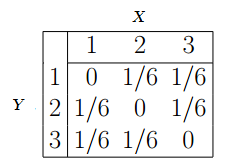# Joint Distribution

## What are Joint Distribution, Moment, and Variation?

### Joint Distribution

Joint distribution is based on joint probability, which can be simply defined as the probability of two events (variables) happening together. These two events are usually coined event A and event B, and can formally be written as:

p(A and B)

Joint distribution, or joint probability distribution, shows the probability distribution for two or more random variables. Hence:

f(x,y) = P(X = x, Y = y)

The reason we use joint distribution is to look for a relationship between two of our random variables. Here, we look at two coins that both have roughly a 50/50 chance of landing on either heads (X) or tails (Y).

 X Y X 25% 25% Y 25% 25%

You can use the chart to determine the probability of a certain event happening by looking at where the two events intersect. Here's another example of a joint distribution table:Source

### Application in Machine Learning

The design of learning algorithms is such that they often depend on probabilistic assumption of the data. Uncertainty is a key concept in pattern recognition, which is in turn essential in machine learning. Being able to make optimal predictions from an incomplete data set by using the data a machine does have is essential in the framework of “smarter” and faster AI.